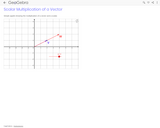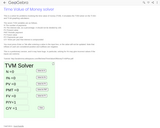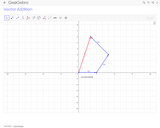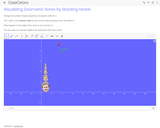Updating search results...

# 24 Results

View
Selected filters:
• GeoGebraConditional Remix & Share Permitted
CC BY-SA
Rating
0.0 stars

Simply shows multiplication of a vector by a scalar. Adjust he slider to adjust the multiple. Drag the point at the end of vector v to change the original vector.

Subject:
Geometry
Mathematics
Material Type:
Simulation
Provider:
GeoGebra
Provider Set:
GeoGebraTube
03/12/2012Conditional Remix & Share Permitted
CC BY-SA
Rating
0.0 stars

This is a solver for problems involving the time value of money (TVM). It emulates the TVM solver on the TI-83+ and TI-84 graphing calculators. Updated 6 November 2011 to work correctly when I% = 0.

Subject:
Finance
Geometry
Mathematics
Material Type:
Simulation
Provider:
GeoGebra
Provider Set:
GeoGebraTube
04/26/2012Conditional Remix & Share Permitted
CC BY-SA
Rating
0.0 stars

Addition of three Vectors, and displays resultant Vectors. Can drag the endPoints of the three different Vectors, but the resultant always starts at the origin.

Subject:
Geometry
Mathematics
Material Type:
Simulation
Provider:
GeoGebra
Provider Set:
GeoGebraTube
03/11/2012Conditional Remix & Share Permitted
CC BY-SA
Rating
0.0 stars

My pre-Calculus students were having trouble visualizing what it meant for a geometric series to converge. They understood the formula aspect and how to calculate the value of a convergent sum, and saw what it meant for numbers adding successively, but I

Subject:
Geometry
Mathematics
Material Type:
Simulation
Provider:
GeoGebra
Provider Set:
GeoGebraTube